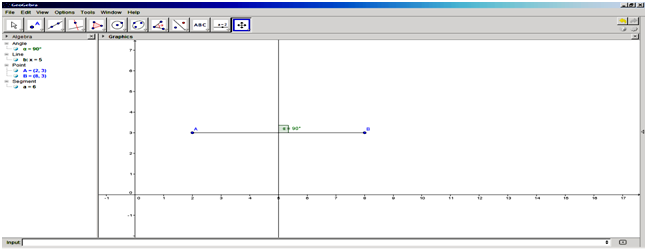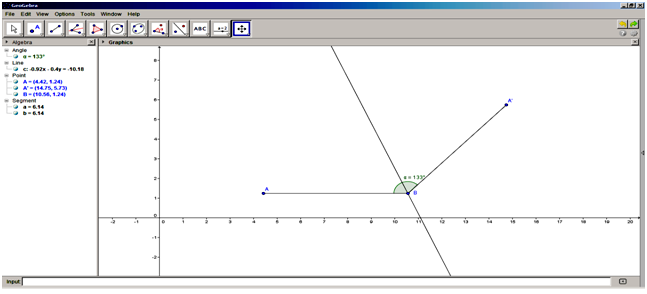## ICT PRACTICALS

### P7

SSC BOARD EXAM ICT Practical P7 - Using Geogebra draw Draw a line segment of length 6.5 cm and draw perpendicular bisector to Line segment.

Practical Name: Using Geogebra draw
i)     Draw a line segment of length 6.5 cm and draw  perpendicular bisector to Line segment
ii)     Draw an angle with measurement 133 and bisect it.

Tools/Software: Computer with Geogebra Software installed.

Procedure:
Draw a line segment of length 6.5 cm and draw  perpendicular bisector to line segment
Using Mouse
1)          Start the Geogebra Software
2)         Click down arrow on Tool No 2 and select “Segment with Fixed Length.” Click anywhere in the Graphics view, it adds a start point A of the Segment and opens a dialog box.
3)         In the dialog box, enter the length as 6.5 and click the OK button
4)         A horizontal segment {a}  of required length will be drawn.
5)         Select the Midpoint or Center tool and click on the segment a to add the midpoint C.
6)         Click on Tool No 3 and select Perpendicular Bisector" Tool and click the segment {a}, a perpendicular bisector line {b} will be created.
7)         Select the Angle tool. Click on the segment a and line c, an angle between them as α = 90° will be shown.

Using keyboard:
1)          Start the Geogebra Software
2)         Click on Input box
3)         Type the commands in the input box as given below:
A=(2,3)
B=(8,3)
Segment(A,B)
PerpendicularBisector[A,B]
Angle[a,b]

Draw an angle with measurement 133 and bisect it.
1)          Select the Segment between two points tool and click at any two points (horizontally) in the Graphics View. This adds two points, A and B and the segment {a}.
2)         Click on Tool No 7 and select ‘Angle with given size’.
In the Graphic area click on point A and then the point B. In the box that comes up enter the measure of the angle that you want e.g., 133o and click on OK button.
3)         The angle α =133o will be drawn.
4)         Select ‘Segment through two points’. Click on the points B followed by A', segment {b} will drawn.
5)         Select Tool 3 and click on ‘Angle Bisector Tool’.
6)         Click on points A followed by B and A’. The required angle bisector line {c} will be drawn.
7)         Save and print your file if required.

Result:  The figures i) and ii) are drawn.Figure No. (i)Figure No. (ii)

#### 1 comment:

1.thank you very much